# Simulation of Smoke

This program simulates a fluid like air or water and show the solution as motion of smoke. Define the velocity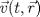and pressure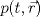in a 3D space. Then the motion of the fluid is determinded by the uncompressible Navier-Stokes equation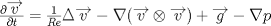.
with the gravitational force g and Reynoldsnumber Re and.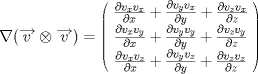In an uncompressible gas, the mass conservation is simply given by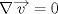.
To solve the Navier Stokes equation a finite difference approach is usedwith a discretized uniform mesh.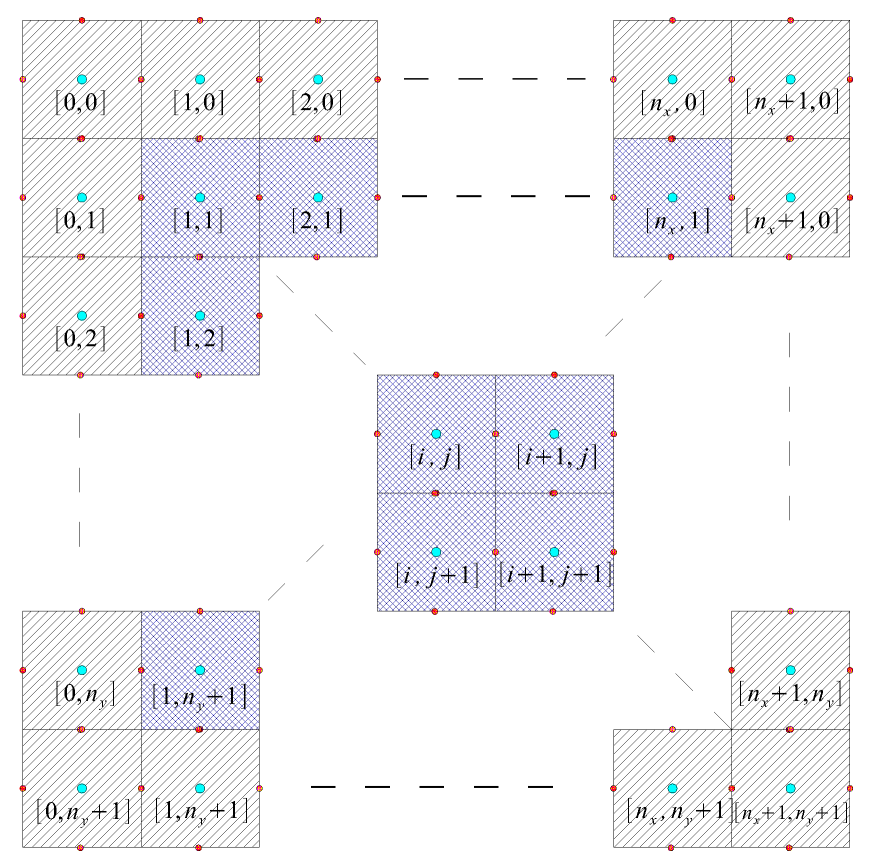. For stability issues I use a staggered grid in which the velocity is defined at the border of the cells.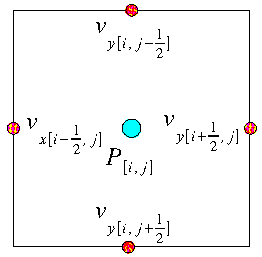.
To solve the time dependence of the Navier-Stokes equation I use the forward Euler scheme with the defined timestep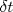. This yields to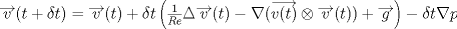and after a substitution of the terms in the bracket with u, the equation becomesPut the last equation into the mass conservation equation and apply the divergence operator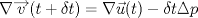You get the poisson equation for the pressure.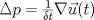.
The finite difference scheme of this equation is solved via the BICGSTAB method.
The output format is a density file used in the raytracer Povray.

Click on the following images to download the videos. This simulation took less than half an hour on an AMD Athlon 1.4 Ghz with a grid size of 60x60x40.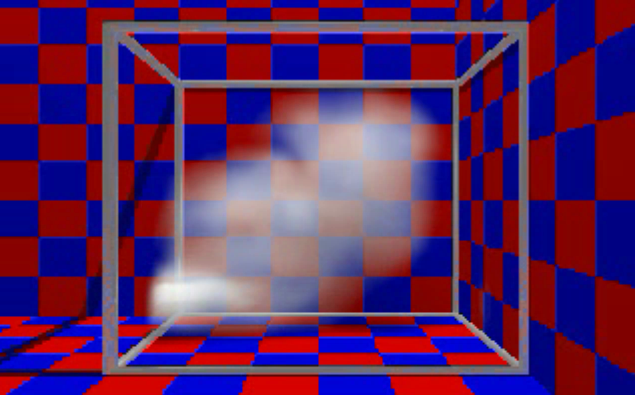The following shows a two phase fluid using the Level Set Method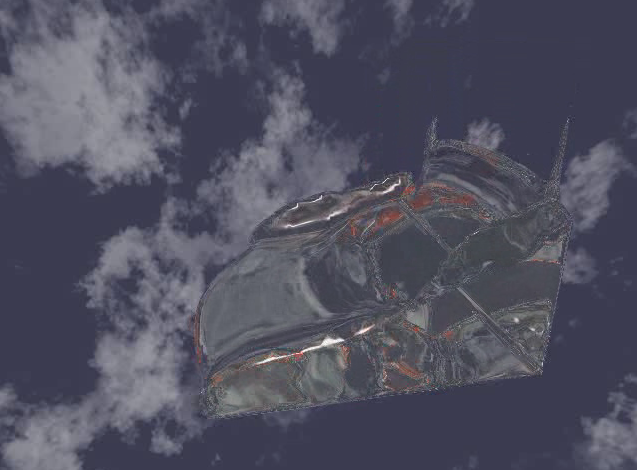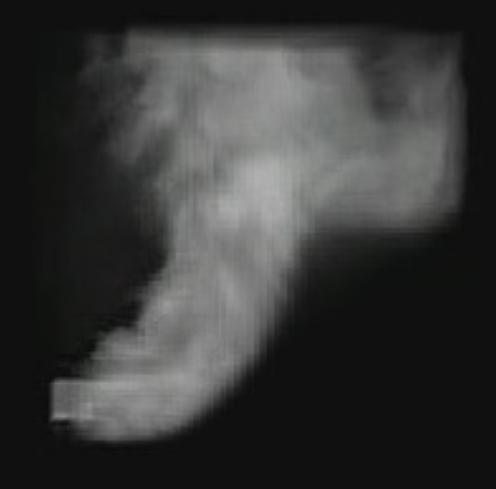and other two
smoke2.avi
smoke3.avi